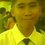# Nth Derivative of Polynomial (Part 2)

Let n be the number of differentiation and b be the exponent of the given term of a polynomial

1. If $n>b$, then the nth derivative of a given polynomial is 0.

Ex. Find the 5th derivative of ${ 2x }^{ 4 }$?

Solution: Since the given n is 5 and b is 4, it adheres that $n>b$, so use the statement 2, thus:

$\frac { { d }^{ 5 } }{ { dx }^{ 5 } } { 2x }^{ 4 }=0$

To prove that this statement is correct, let's use the repeated differentiation method.

$y={ 2x }^{ 4 }$

$\frac { dy }{ dx } ={ 2(4)x }^{ 4-1 }={ 8x }^{ 3 }$

$\frac { { d }^{ 2 }y }{ { dx }^{ 2 } } ={ 8(3)x }^{ 3-1 }={ 24x }^{ 2 }$

$\frac { { d }^{ 3 }y }{ { dx }^{ 3 } } ={ 24(2)x }^{ 2-1 }={ 48x }^{ 1 }=48x$

$\frac { { d }^{ 4 }y }{ { dx }^{ 4 } } ={ 48(1)x }^{ 1-1 }={ 48x }^{ 0 }=48$

$\frac { { d }^{ 5 }y }{ { dx }^{ 5 } } =0$

Note: If we find the 6th, 7th or either the 1000th derivative of ${ 2x }^{ 4 }$, it will give also a derivative of 0 since n is greater than b.Note by Merzel Mark Guilaran
6 years, 7 months ago

This discussion board is a place to discuss our Daily Challenges and the math and science related to those challenges. Explanations are more than just a solution — they should explain the steps and thinking strategies that you used to obtain the solution. Comments should further the discussion of math and science.

When posting on Brilliant:

• Use the emojis to react to an explanation, whether you're congratulating a job well done , or just really confused .
• Ask specific questions about the challenge or the steps in somebody's explanation. Well-posed questions can add a lot to the discussion, but posting "I don't understand!" doesn't help anyone.
• Try to contribute something new to the discussion, whether it is an extension, generalization or other idea related to the challenge.

MarkdownAppears as
*italics* or _italics_ italics
**bold** or __bold__ bold
- bulleted- list
• bulleted
• list
1. numbered2. list
1. numbered
2. list
Note: you must add a full line of space before and after lists for them to show up correctly
paragraph 1paragraph 2

paragraph 1

paragraph 2

[example link](https://brilliant.org)example link
> This is a quote
This is a quote
    # I indented these lines
# 4 spaces, and now they show
# up as a code block.

print "hello world"
# I indented these lines
# 4 spaces, and now they show
# up as a code block.

print "hello world"
MathAppears as
Remember to wrap math in $$ ... $$ or $ ... $ to ensure proper formatting.
2 \times 3 $2 \times 3$
2^{34} $2^{34}$
a_{i-1} $a_{i-1}$
\frac{2}{3} $\frac{2}{3}$
\sqrt{2} $\sqrt{2}$
\sum_{i=1}^3 $\sum_{i=1}^3$
\sin \theta $\sin \theta$
\boxed{123} $\boxed{123}$

Sort by:

Do you know how to prove the original statement is true?

Staff - 6 years, 7 months ago

No. I lack of proofs of it but for sure the statement is true because there are many times that I try to use some polynomial expressions and find the most higher order derivative to find the derivative of the given polynomial and the result is correct and adheres the given statement. I will try my best to prove this statement true. thanks

- 6 years, 7 months ago